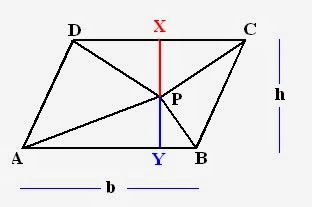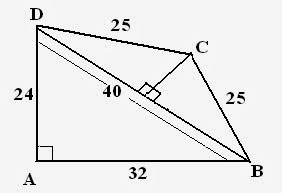# Important questions of Geometry for SSC CGL Tier I In my previous session, I have discussed some concepts related to triangles. Today I will discuss sReeves 2021-02-16 Answered
Important questions of Geometry for SSC CGL Tier I In my previous session, I have discussed some concepts related to triangles. Today I will discuss some important questions of Geometry which used to appear in SSC exams. Generally, questions asked from this section are based on properties of various shapes like lines, angles, triangles, rhombus, circles etc.
You can still ask an expert for help

• Questions are typically answered in as fast as 30 minutes

Solve your problem for the price of one coffee

• Math expert for every subject
• Pay only if we can solve itquestion2answer

## Important Examples

Example1: ABCD  is a cyclic quadrilateral. AB and CD are produced to meet P. If angle ADC = ${70}^{o}$and $\mathrm{\angle }$$DAB={60}^{o}$, then what will be angle $PBC+\mathrm{\angle }PCB$?

Solution: First step: Make an appropriate figure using statements provided in  questions.• Cyclic quadrilateral has all its vertices on circle and sum of all angles is ${360}^{\circ }$.
• Sum of opposite angles = ${180}^{\circ }$.
• External angle = Opposite internal angle.

Therefore, $\mathrm{\angle }$ $PBC={70}^{o}$
$\mathrm{\angle }$$PCB={60}^{o}$

So, $PBC+PCB={130}^{0}$

See its very simple question, if you know the properties of geometry. Let's try more examples

Example2: ABCD is a parallelogram and P is any point within it. If area of parallelogram is 20 units, then what will be the sum of areas of triangle PAB and PCD?

Solution: According to the question, figure will be as follows:Properties for this question:

• Area of parallelogram = Base times Height.
• Area of triangle = 1/2 Base times altitude.

Given that: Area of parallelogram = 20
i.e. Base times Height = 20
$⇒b×h=20$

To find =  Area of ( PAB +PCD)
$⇒\left(1/2\right)b×\left(PY\right)+\left(1/2\right)b×\left(PX\right)$ ( See Figure)

$⇒1/2b\left(PX+PY\right)$

$⇒1/2bh$
⇒(1/2) $×$ 20
$⇒10units$.

Example3: ABCD is a cyclic trapezium with AB parallel DC and AB diameter of circle. If $\mathrm{\angle }$$CAB={30}^{o}$, then $\mathrm{\angle }ADC$ will be?

Solution: According to ques, figure will be as follows:• Angle subtended by diameter is always ${90}^{\circ }$.
• Sum of angles of triangle = ${180}^{o}.$
• Sum of opposite angles = ${180}^{o}$

$\mathrm{\angle }$ $ACB={90}^{o}$
$\mathrm{\angle }$ $BAC={30}^{o}$

Therefore,

$\mathrm{\angle }$ $ABC={60}^{o}$
angle $ABC+\mathrm{\angle }$ $ADC={180}^{o}⇒{60}^{o}+ADC={180}^{o}$$\mathrm{\angle }$ $ADC={120}^{o}$
Example4: Two side of plot measures 30m and 22m and angle between them is ${90}^{o}$. The other two sides measures 24m and the three remaining angles are not right angles. Find the area of plot.
Solution: Figure becomes: Center point of BD = OABD is a right angle triangle, therefore, Pythagoras theorem followed.
$\left(BD{\right)}^{2}=\left(AB{\right)}^{2}+\left(AD{\right)}^{2}⇒BD=40$
Given that, $BC=CD$ Therefore, line drawn from C to BD given right angles Also, this will lead to DO = BO
So, $DO=BO=20$
Similarly, using Pythagoras theorem, $OC=15m$

Now, Area of triangles $\left(ABD+BOC+COD\right)⇒1/2\left(24×32\right)+2\left(\left(1/2\right)20×15\right)⇒684$ ${m}^{2}$